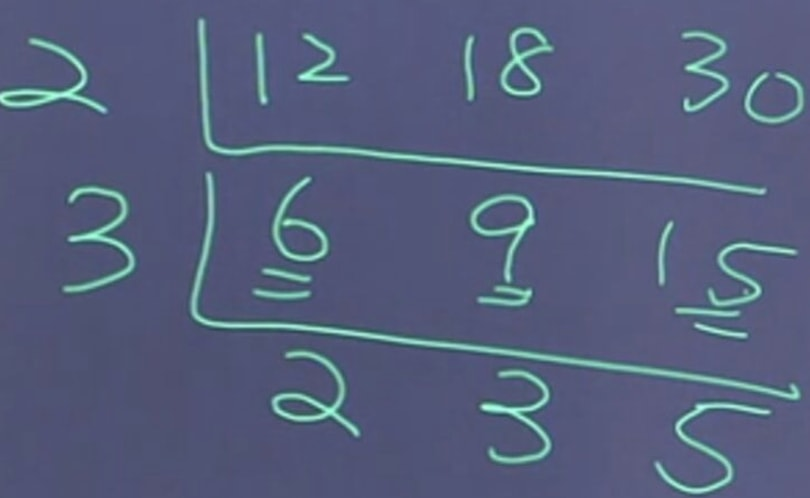# Factor by taking out the greatest common factor

## How to factor a polynomial

What happens when you are asked to factor a polynomial? It's actually quite similar to what you do when you factor numbers. You'll have to find numbers that you can divide out from the polynomials evenly. Unlike working with just numbers, in polynomials you have to divide numbers out of terms rather than just a single number.

## Finding factors of polynomials

So how do you go about finding what the factors are in a polynomial? You'll have to learn to spot what can be factored out of every term. This is the common factor.

In the past, when you're asked to simplify expressions, you'd have to distribute numbers into terms in parentheses. For example, in 4(x+2), you'd evaluate it as 4x+8. When we're factoring out terms, you'll have to do the opposite of that! Other than just learning to look at a set of polynomials and identifying the factor, you can also use the method of finding the greatest common factor. Let's learn this first step to factoring polynomials.

## What is the greatest common factor

In order to find the greatest common factor (GCF), you'll have to find the prime factors for each of the numbers you are working with. Then you'll multiply the factors that all the numbers have in common.

So for example, if you had the numbers 10 and 5 and you had to find their common factor, you'd first tackle the 10, and then the 5 as follows:

$\footnotesize2\frac{)10}{5}$

$\footnotesize5\frac{)5}{1}$

In both of these, you see that you have 5. Since there's only 1 factor in common, you don't have to multiply the 5 by anything else. Therefore, you've found that 5 is the biggest numbers that divides evenly into 10 and 5. If you don't find any common factors, then your GCF will be 1.

This is the method you'll employ in order to find the factors of polynomials. Follow along as we solve the upcoming example questions to see how the GCF is used when factoring expressions.

## Example problems

Question 1:

Factor $12p^{7} - 18p^{2} - 30$

Solution:

Look for the greatest common factor of this polynomial using long division. You can do each of the terms separately or you can do them altogether like what we did here:GCF of multiple numbers

The GCF of this polynomial is found through multiplying the common factors from all three of the numbers together. This means you'll get:

(2)(3)=6

We then factor out 6 from each term of the polynomial, and we'll get the final answer of:

$6[2p^{7} - 3p^{2} - 5]$

Question 2:

Factor $10z(x + 2y) - 6(x + 2y)$

Solution:

Firstly, let us look for the common factors of the polynomial. When you first look at the numbers, you'll likely spot out that the common factor of 10 and 6 is 2.

Another common factor is (x+2y). So, we factor out both and we'll get the final answer of:

(2)(x + 2y)[5z - 3]

If you ever need to double check your answer when trying to find the common factors of polynomials, try out this online GCF calculator. It will help you be sure of your answers when you factor more complex polynomials. As always, do remember that the calculator should only be used to check your answers rather than doing the questions for you!

Ready to move on? Learn how to complete the square in quadratic functions, convert quadratic functions from general to vertex form, and solve quadratic equations by factoring or by completing the square.

### Factor by taking out the greatest common factor

To factor means to take out a common factor out from the expressions. In this lesson, we will try to do it by determining the greatest common factor among the terms of the expressions, and factor it out from each term.

#### Lessons

• Introduction
review "factoring"

• 1.
a)
$12{p^7} - 18{p^2} - 30$

b)
$10z\left( {x + 2y} \right) - 6\left( {x + 2y} \right)$